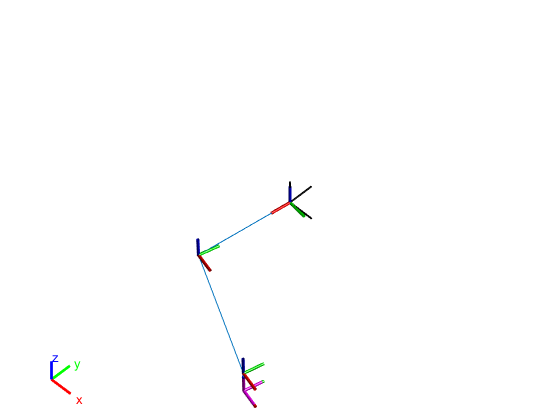• MATLAB 2017b Parallel Computing Toolbox官方教程，包含用户手册和系统管理
• MATLAB Neural Network Toolbox官方教程，包含入门教程，用户手册和参考手册
• PST consists of a set of coordinated MATLAB m-files which model the power system components necessary for power system power flow and stability studies. The toolbox comes with the m-files, demo ...
• matlab-mapping toolbox-提取自r2019b,解压后放到matlab目录下的toolbox中，并在预设目录中添加他和子目录，最后在预设-常规中点击更新工具箱路径缓存即可
• 利用MATLAB和Robot toolbox 做的一个简单的三轴机器人GUI，可以实现正逆运动学演示和内部teach（）函数类似。teach函数是由slider控制的，这个GUI是用可编辑文本输入数字完成。
• Deep Learning Toolbox™提供了一个框架，用于设计和实现具有算法，预训练模型和应用程序的深度神经网络。您可以使用卷积神经网络（ConvNets，CNN）和长期短期记忆（LSTM）网络对图像，时间序列和文本数据进行分类和...
• MATLAB Image Processing Toolbox官方教程，包含用户手册和参考手册
• Matlab官网5G toolbox介绍信息汇总 对官网关于matlab2020a 5G工具箱信息的总结汇总
• Matlab工具箱（GRAMAT）包含组开源函数，用于处理GRACE 2级球面谐波系数乘积。 GMT中的数据处理功能包括：（1）去除SH系数以去除“从北到南”的条纹和高斯平滑；（2）球谐分析和合成；（3）分析和减少GRACE派生的...
• Matlab Communications System Toolbox 2016版用户手册。包含 Getting started, user's guide, Reference
• MATLAB Text Analytics Toolbox官方教程，包含实例和参考手册
• Matlab Robotics Toolbox，介绍 Matlab Robotics Toolbox函数详细使用方法，包含 Matlab Robotics Toolbox所有函数
• MATLAB 2017b Communications System Toolbox官方教程，包含入门教程，用户手册和参考手册
• Matlab Robotics Toolbox款功能强大的机器人工具箱软件，由于Matlab版本的升级，目前，可以使用的最新版为V10.2，较之前的版本而言，函数发生了一定变化，如，将drivebot()替换为teach()等等。 工具箱的安装：...
• 该工具箱包含组标准的MATLAB函数，用于分析和显示气候数据。 这些函数计算效率高，易于使用，并且附带了许多教程，这些教程不仅描述了如何使用CDT函数，而且还提供了有关如何在地球科学过程的背景下解释结果的指南...
• matlab2014版本的RF工具箱的使用说明文档
• 【基于Matlab Robotics Toolbox的Dobot机械臂运动规划】系列文章是我在学习robotics toolbox中所做工作的记录，方便自己...所以我选择使用app designer做一个图形界面。 首先做正运动学部分。 在设计视图里把组件拖
• MATLAB Statistics Toolbox R2012b pdf document for you student travel
• Documentation for Matlab ToolboxMaltab
• MATLAB工具箱大全- 张量工具箱Tensor Toolbox
• MATLABLaydataToolbox-MATLAB-Laydata Toolbox.rar MATLAB-Laydata Toolbox.zip
• 附有安装说明和说明文档《robotics toolbox for matlab release 9(version,9.10)》，希望能够给大家带来方便
• MATLAB Instrument Control Toolbox
• 是对robotics toolbox函数的介绍，靠谱，给力，来之不易，现在分享给大家，希望大家喜欢，matlab-robotics-toolbox
• GRACE Matlab Toolbox (GRAMAT) 包含组用于处理 GRACE 2 级球谐系数乘积的开源函数。 GMT 中的数据处理功能包括：(1) 去除 SH 系数以去除“南北向”条纹和高斯平滑，(2) 球谐分析和合成，(3) 分析和减少 GRACE ...
• 这里写自定义目录标题前记创建一个机器人并显示刚体树类功能快捷键合理的创建标题，有助于目录的生成如何改变文本的样式插入链接与图片如何插入一段漂亮的代码片生成一个适合你的列表创建一个表格设定内容居中、居左...


这里写自定义目录标题
前记创建一个机器人并显示创建机器人模型创建关节和连杆刚体与关节刚体关节Transform-相邻坐标系的变换将关节添加到刚体上

显示机器人模型写在最后

前记
一开始打算使用petet corke的matlab robotics toolbox来建立一个机器人模型，但是不知道什么原因，在使用逆动力学和显示时总是报错。 具体配置是r2019b+10.2版本的工具箱，但是在r2019a版本则可以正确运行，在各种查阅无果之后，只能使用matlab官方的robotics system toolbox来实现。
创建一个机器人并显示
参考代码来自matlab官方工具箱示例，这里主要对参考代码中的函数（或者说类？）进行解析，当然查api肯定是坠吼的，然而我懒得总是翻英文文档，所以写这篇文章做一个提示。
创建机器人模型
robot = rigidBodyTree('DataFormat','column','MaxNumBodies',3);

这行代码实例化了一个刚体树类，‘DataFormat’为‘column’，也就是说数据是以行读入的，如果为‘row’那么则需要对向量进行转置后才能读入数据；'MaxNumBodies’参数为3，表示该刚体树模型不包括基的最大刚体数为3。 原文的说明如下

Number of bodies in the robot model (not including the base), returned as an integer.

创建关节和连杆
刚体与关节
刚体
L1 = 0.3;
L2 = 0.3;

body = rigidBody(name)

name为刚体的名称，在默认情况下，刚体对象的属性Joint为fixed，即固定关节；Mass默认为1kg，CenterofMass即质心位置为[0 0 0]
关节
joint = rigidBodyJoint('joint1', 'revolute');


关节对象采用rigidBodyJoint函数创建，语法为
joint - rigidBodyJoint(name,type)

第二个参数有三种类型，分别是’fixed’固定关节、'revolute’转动关节和’prismatic’移动关节。 关节的属性： (1)PositionLimits— 关节向量的位置限制 位置限制[min max] fixed没有限制；revolute默认为[-pi pi]；prismatic默认为[-0.5 0.5](单位为米 (2)HomePosition— 关节的初始位置 HomePostion为一个标量，默认为0，对于转动关节为初始角度，对于移动关节为沿关节轴的移动距离(单位仍是m） (3)JointAxis— 关节轴 JointAxis为一个向量[x y z]，默认为NaN (4)JointToParentTransform和ChildToJointTransform 这两个属性没太弄懂，官方在刚体树模型里有图文解释。
Transform-相邻坐标系的变换
setFixedTransform(joint,trvec2tform([0 0 0]));
joint.JointAxis = [0 0 1];

这里的transform应该是和关节的属性JointToParentTransform和ChildToJointTransform有关，具体我仍是不太清楚（爬）。 主要介绍一下setFixedTransform函数 (1)语法 seFixedTransform有三种调用方式，分别是tform齐次变换，标准dh参数，modified dh参数。
setFixedTransform(jointObj,tform)
setFixedTransform(jointObj,dhparams,"dh")
setFixedTransform(jointObj,mdhparams,"mdh")

tform是坐标系变换的4x4矩阵，具体形式为

[

r

11

r

12

r

13

p

x

r

21

r

22

r

23

p

y

r

31

r

32

r

33

p

z

0

0

0

1

]

\begin{bmatrix} r_{11}&r_{12} &r_{13} &p_{x} \\ r_{21}&r_{22} &r_{23} &p_{y} \\ r_{31}& r_{32}& r_{33}&p_{z} \\ 0& 0& 0& 1 \end{bmatrix}

标准dh法和mdh法的参数形式均为 [a alpha d theta]，关于这两种方法有何不同，请跳转 建立DH模型的三种方法以及区别 注意，dh法的最后一个元素theta将会被忽略，因为具体的theta值取决于关节姿态;个人认为这是对于转动关节来说的，因为这段文字源于matlab官方在建立puma560机器人模型时的描述，而puma560并没有移动关节；对于移动关节则应该是忽略第三个参数d。
将关节添加到刚体上
body.Joint = joint;

addBody(robot,body,parentname)

robot是一个刚体树模型，body是你想要添加的刚体对象，parentname是一个字符串，为body的父对象的名称，也可以理解为与body相连的连杆。
%Add 'link2' body with 'joint2' joint.

joint = rigidBodyJoint('joint2','revolute');
setFixedTransform(joint, trvec2tform([L1,0,0]));
joint.JointAxis = [0 0 1];
body.Joint = joint;
%Add 'tool' end effector with 'fix1' fixed joint.

body = rigidBody('tool');
joint = rigidBodyJoint('fix1','fixed');
setFixedTransform(joint, trvec2tform([L2, 0, 0]));
body.Joint = joint;
%Show details of the robot to validate the input properties. The robot should have two non-fixed joints for the rigid bodies and a fixed body for the end-effector.

显示机器人模型
showdetails(robot)

showdetails()会显示机器人关节的详情，输出示例如下（注：这并不是官方例程的输出）
--------------------
Robot: (4 bodies)

Idx    Body Name    Joint Name    Joint Type    Parent Name(Idx)   Children Name(s)
---    ---------    ----------    ----------    ----------------   ----------------
--------------------

在空间中显示机器人模型图片
show(robot);
axis([-0.5 0.5 -0.5 0.5 -0.5 0.5])
axis off
%获取机器人的关节姿态
config = randomConfiguration(robot);
%将机器人的关节随机初始化
tform = getTransform(robot,config,'tool','base')
%获取tool坐标系相对于base的变换矩阵

效果如下（仍然不是官方示例）写在最后
这里只截取了官方例程的一部分，在原有例程中还有逆动力学部分，用来实现对于一个圆的轨迹追踪，这里直接并没有提及，要想了解剩余的部分，可以跳转具有逆运动学的2维轨迹追踪或者官方例程 小白刚入坑matlab，如果有错误之处，还请多多指正。
展开全文• 来源于MATHWORKS,感谢白途思童鞋点拨。http://www.cnblogs.com/begtostudy/archive/2010/08/02/1790386.html...

# matlab的一个toolboxmatlab 订阅